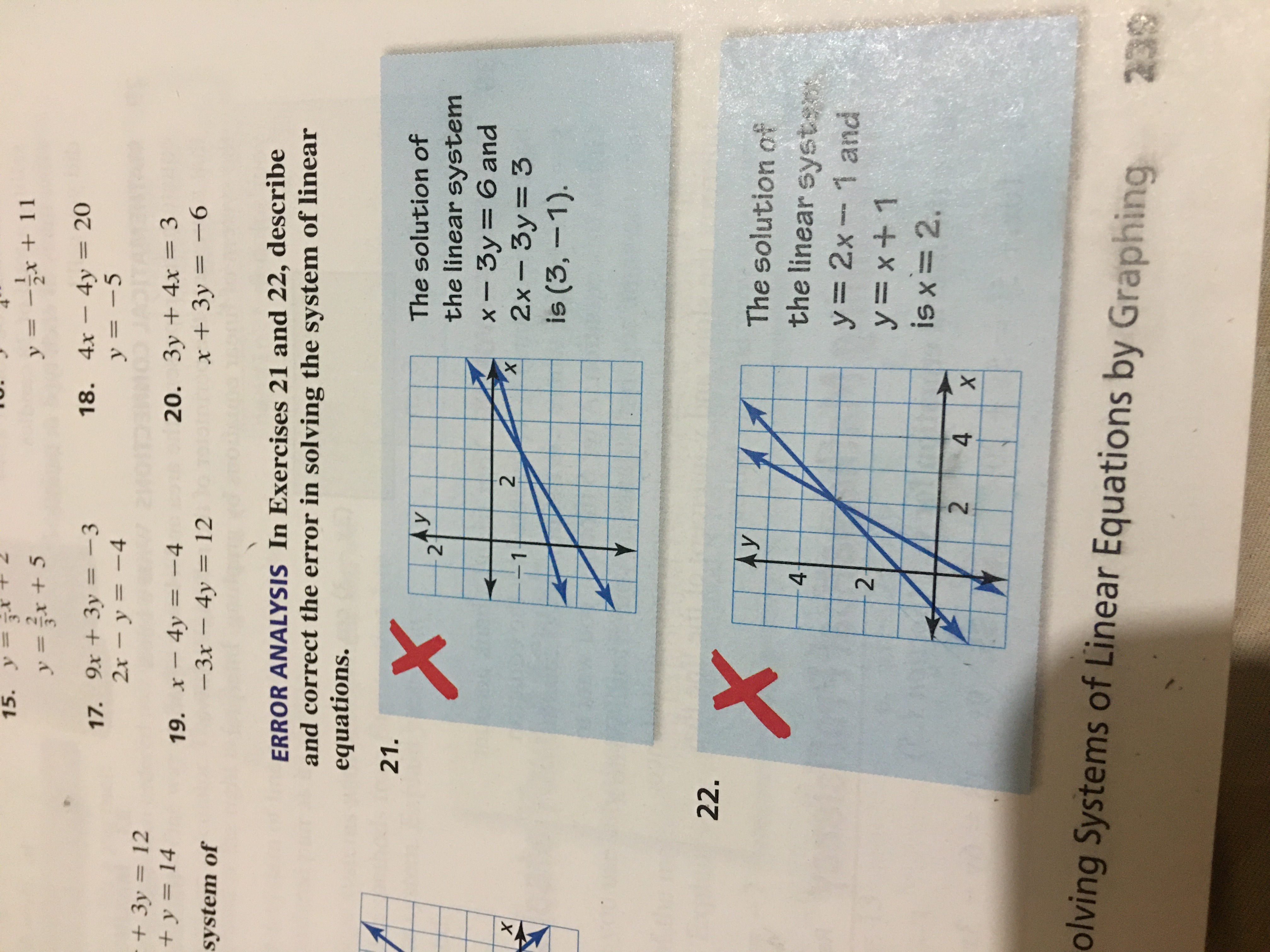# 15. y3y = -r + 11y =r +518. 4x4y = 20y = -517. 9x+3y= -32x- y-4+3y 1220. 3y +4x = 3x +3y=-6+y= 1419. x4y -4-3x - 4y 12system ofERROR ANALYSIS In Exercises 21 and 22, describeand correct the error in solving the system of linearequations.21.The solution ofAy2the linear systemx-3y= 6 and2x- 3y 3is (3, -1).2хх122.XAyThe solution ofthe linear syst4-y= 2x1 and2y=x+ 1is x 2.24Xolving Systems of Linear Equations by Graphing239

Question
8 views

I need help with number 22. I can't seem to find the mistake.help_outlineImage Transcriptionclose15. y3 y = -r + 11 y =r +5 18. 4x4y = 20 y = -5 17. 9x+3y= -3 2x- y-4 +3y 12 20. 3y +4x = 3 x +3y=-6 +y= 14 19. x4y -4 -3x - 4y 12 system of ERROR ANALYSIS In Exercises 21 and 22, describe and correct the error in solving the system of linear equations. 21. The solution of Ay 2 the linear system x-3y= 6 and 2x- 3y 3 is (3, -1). 2 х х 1 22. X Ay The solution of the linear syst 4- y= 2x1 and 2 y=x+ 1 is x 2. 2 4 X olving Systems of Linear Equations by Graphing 239 fullscreen
check_circle

Step 1

The given linear ...

### Want to see the full answer?

See Solution

#### Want to see this answer and more?

Solutions are written by subject experts who are available 24/7. Questions are typically answered within 1 hour.*

See Solution
*Response times may vary by subject and question.
Tagged in

### Algebra